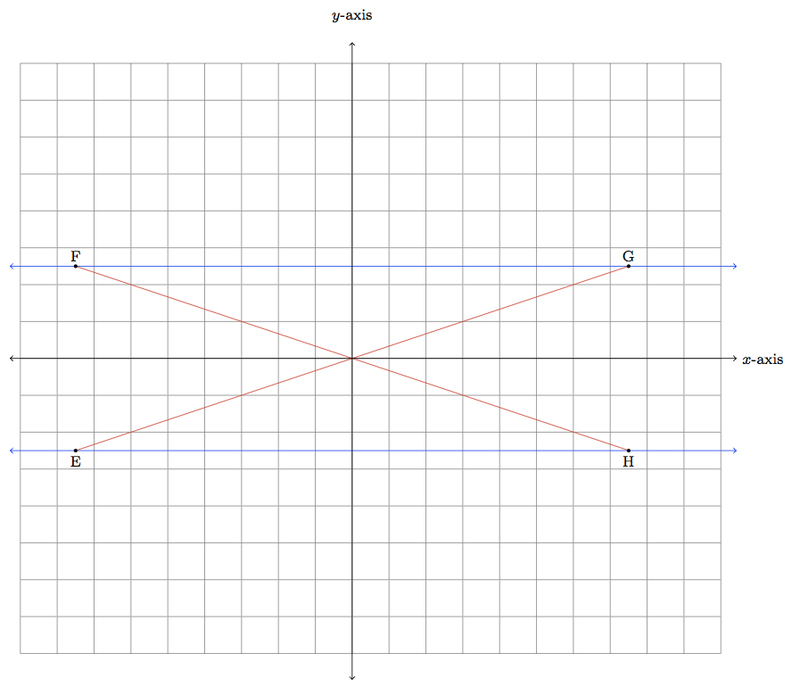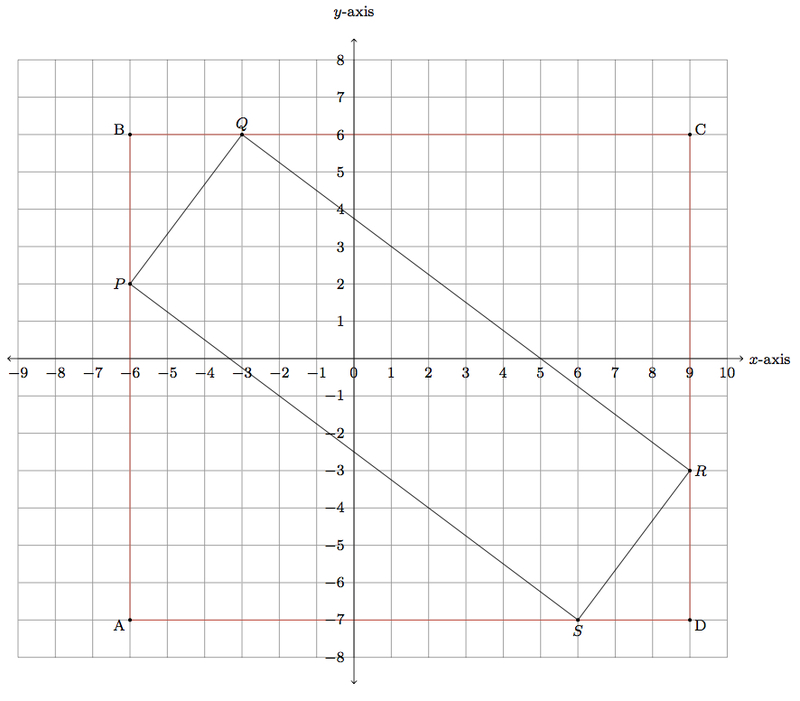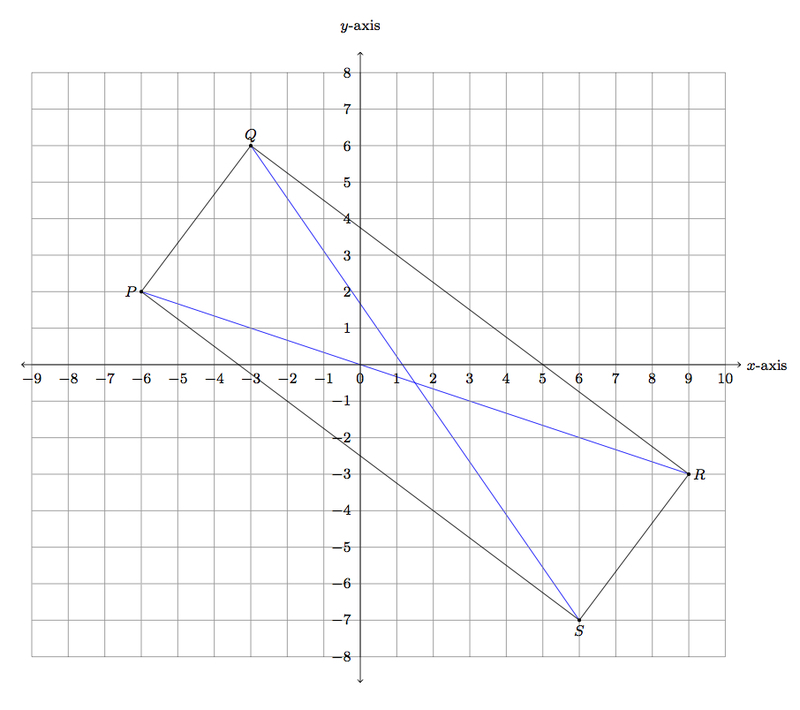# Is this a rectangle?

Alignments to Content Standards: 8.G.A 8.G.B G-CO.B G-GPE.B G-SRT.B.5

Is the quadrilateral with vertices $(-6, 2)$, $(-3,6)$, $(9, -3)$, $(6,-7)$ a rectangle? Explain.

## IM Commentary

The goal of this task is to provide an opportunity for students to apply a wide range of ideas from geometry and algebra in order to show that a given quadrilateral is a rectangle. Creativity will be essential here as the only given information is the Cartesian coordinates of the quadrilateral's vertices. Using this information to show that the four angles are right angles will require some auxiliary constructions. Students will need ample time and, for some of the methods provided below, guidance. The reward of going through this task thoroughly should justify the effort because it provides students an opportunity to see multiple geometric and algebraic constructions unified to achieve a common purpose. The teacher may wish to have students first brainstorm for methods of showing that a quadrilateral is rectangle (before presenting them with the explicit coordinates of the rectangle for this problem): ideally, they can then divide into groups and get to work straightaway once presented with the coordinates of the quadrilateral for this problem.

This task has been worded to support a wide range of approaches which help link eighth grade geometry standards with high school geometry (and algebra) standards. It is important that different methods of solution be considered because this reveals the connections between different standards. In order to solve this problem, students must show that the four angles in the quadrilateral are all right angles. One method to achieve this, at the eighth grade level, is with the Pythagorean theorem and its converse. Also at the eighth grade level, rigid motions of the plane can be applied to the quadrilateral so that its angles are shown to be congruent to angles made by horizontal and vertical grid lines. At the high school level, one way to show that an angle is a right angle is to make algebraic calculations of slopes of the lines containing the two rays of the angle. A second method produces several auxiliary triangles and uses the important notion of similarity. For this last solution, the teacher may wish to provide extra prompting: drawing the coordinate rectangle circumscribing the given quadrilateral is a starting point but some encouragement to study the four triangles left when the given quadrilateral is removed may also be necessary. Two final solutions have been presented, one making use of the $SSS$ congruence criterion for triangles and the final one using a criterion for when a parallelogram is a rectangle.

One of the advantages to open-ended tasks like this one is that they allow for multiple, creative solutions and, in so doing, show how the different strands of the curriculum are related. This task supports the transformational approach to geometry adapted in the CCSS in a very strong way: the second solution uses rotations and translations in a fundamental way while the fourth solution requires an understanding of similar triangles and consequently of dilations of the plane. At the same time, it gives an opportunity to relate these new ideas to classical approaches using the Pythagorean Theorem and slopes of lines.

This task is intended for instructional purposes only. The open-ended nature of the task makes it ideally suited for extended work and discussion, including sharing of ideas and different approaches. Two of the solutions are appropriate for the eighth grade while the other four require knowledge of high school content so ideally students will work on this both in middle school and then later in high school.

Teachers working with 8th grade students may choose to provide scaffolded prerequisite tasks that present the coordinates for four vertices of a quadrilateral with its center at (0,0) in order to employ a single rigid motion (rotation) and then a separate set of coordinates for four vertices forming a quadrilateral with side parallel to the grid lines. Students could then consider both of these prerequisite tasks and strategies useful for solving them when presented with this task item as written. There is also a more directive version of this task that asks students to use the Pythagorean theorem: 8.G A rectangle in the coordinate plane

The solution 6 is the high school version of solution 2. The rest of the solutions represent different approaches as they are conceptually very different from one another.

## Solutions

Solution: Pythagorean theorem and its converse (8.G.7 and 8.G.8)

Below is a picture of the quadrilateral, labelled $PQRS$, with the two diagonals added, along with points $M$ and $N$ which will help to calculate the length of the two diagonals $\overline{PR}$ and $\overline{QS}$ using the Pythagorean theorem.We can find the lengths of $\overline{PR}$ and $\overline{QS}$ applying the Pythagorean theorem to the right triangles $PMR$ and $SNQ$.

\begin{align} |PR|^2 &= |PM|^2 + |MR|^2 \\ |SQ|^2 &= |SN|^2 + |NQ|^2 \end{align}

The lengths on the right can all be calculated by using the coordinates. For example $|PM| = 5$ and $|MR| = 15$ since $P = (-6,2)$, $M = (-6,-3)$, and $R = (9,-3)$. Similarly $|SN| = 13$ and $|NQ| = 9$. Plugging these values into the above equations we find $$|PR| = |SQ| = \sqrt{250}.$$

To show that $PQRS$ is a rectangle, we need to show that the four angles are right angles. To do this, we use the converse of the Pythagorean theorem which we will apply to triangles in the picture below:For example to show that angle $PQR$ is a right angle, we will show that $|PR|^2 = |PQ|^2 + |QR|^2$. We already have found $|PR|^2$ above, $|PR|^2 = 250$. To find $|PQ|^2$ we apply the Pythagorean theorem to $\triangle PQF$, a right triangle with hypotenuse $\overline{PQ}$. We have $$|PQ|^2 = |QF|^2 + |PF|^2.$$ Since $|QF| = 3$ and $|PF| = 4$ we find $|PQ|^2 = 9 + 16 = 25$. Similarly to find $|QR|$ we apply the Pythagorean theorem to $\triangle QRG$, a right triangle with hypotenuse $\overline{QR}$. We have $$|QR|^2 = |QG|^2 + |RG|^2 = 12^2 + 9^2 = 225.$$ Putting together everything we have found we have

\begin{align} |PR|^2 &= 250 \\ &= 225 + 25 \\ &= |QR|^2 + |PQ|^2. \end{align}

By the converse of the Pythagorean theorem applied to $\triangle PQR$, $\angle PQR$ is a right angle.

This argument can be repeated four times to show that the other four angles in quadrilateral $PQRS$ are right angles. For example, to show that angle $QRS$ is a right angle, we calculate $|QR|$ applying the Pythagorean theorem to $\triangle QGR$, then we find $|RS|$ applying the Pythagorean theorem to $\triangle RHS$. We found $|SQ|^2$ above and so we can conclude that angle $QRS$ is a right angle by verifying that $|SQ|^2 = |QR|^2 + |RS|^2$.

Solution: Rigid motions of the plane (8.G.2 and 8.G.3)

The goal here is first to translate the quadrilateral so that it is centered at $(0,0)$ and then apply a rotation about $(0,0)$ so that the lines containing the sides of the rectangle are parallel to the grid lines. Notice that $(-3,6)$ is the point of the rectangle with the largest $y$ coordinate while $(6,-7)$ is the point with the smallest $y$ coordinate. The point with the largest $x$ coordinate is $(9,-3)$ while the point with the smallest $x$ coordinate is $(-6,2)$. We need to translate to the left by $1.5$ units and up by $0.5$ units to center this rectangle around $(0,0)$. The vertices of the translated rectangle are $(-7.5,2.5)$, $(-4.5,6.5)$, $(7.5,-2.5)$, $(4.5,-6.5)$. The translated rectangle, with vertices $A = (-7.5,2.5)$, $B = (-4.5,6.5)$, $C = (7.5,-2.5)$, $D = (4.5,-6.5)$ is pictured below:Notice that the quadrilateral is a parallelogram: we can see this by computing the slopes of the lines containing opposite sides of the parallelogram. For example $$\text{slope}\left(\overleftrightarrow{AB}\right) = \frac{2.5-6.5}{-7.5 -(-4.5)} = \frac{-2.5-(-6.5)}{7.5 - 4.5} = \text{slope}\left( \overleftrightarrow{CD}\right)$$ which we can see without evaluating as the right hand expression is the left hand expression multiplied by $\frac{-1}{-1} = 1$. Similarly $$\text{slope}\left(\overleftrightarrow{BC}\right) = \frac{6.5-(-2.5)}{-4.5-7.5} = \frac{-6.5-2.5}{4.5-(-7.5)} = \text{slope}\left(\overleftrightarrow{DA}\right).$$ Notice that the diagonals $\overline{AC}$ and $\overline{BD}$ of quadrilateral $ABCD$ both pass through $(0,0)$. This is because the coordinates of $C$ are opposite the coordinates of $A$ and similarly for $B$ and $D$. The diagonals of $ABCD$ are also congruent as can be seen, for example by applying the Pythagorean theorem to find the distance from $(0,0)$ to $A$, $B$, $C$, and $D$. For example, the distance from $A$ to $(0,0)$ is $\sqrt{7.5^2 + 2.5^2}$. This is the same as $\sqrt{4.5^2 + 6.5^2}$ which is the distance from $B$ to $(0,0)$.

Suppose we rotate $ABCD$ about $(0,0)$ until we find a new quadrilateral $EFGH$ with $\overleftrightarrow{FG}$ and $\overleftrightarrow{EH}$ both parallel to the $x$-axis. The diagonals $\overline{EG}$ and $\overline {FH}$ still meet at $(0,0)$ and are congruent as shown below:Looking at the picture, suppose we fix $E$ and $G$ and then choose $x$ and $y$ with $x$ on the upper blue line, $y$ on the lower blue line so that $(0,0)$ is contained in $\overline{xy}$. If $x$ is to the left of $F$ or to the right of $G$, then $|xy| \gt |EG|$. If $x$ is between $F$ and $G$ then $|xy| \lt |EG|$. So if $|xy| = |EG|$ then $x = F, y = H$ where $EFGH$ is a rectangle.

Solution: Slopes of lines (G.GPE.4 and G.GPE.5)

We label the vertices of the quadrilateral as follows: $P = (-6,2)$, $Q = (-3,6)$, $R = (9,-3)$, $S = (6,-7)$:The idea of this approach is to consider the lines containing the four sides of quadrilateral $PQRS$ and show, using the slope criterion, that they meet in right angles. Recall that the slope criterion says that if line $L_1$ has slope $s_1 \neq 0$ and line $L_2$ has slope $s_2 = \frac{-1}{s_1}$ then $L_1$ and $L_2$ are perpendicular (at the single point where they meet). We calculate the slopes of the lines containing adjacent vertices of $PQRS$:

\begin{align} \text{slope}\left(\overleftrightarrow{PQ}\right) &= \frac{2-6}{-6-(-3)} = \frac{-4}{-3}\\ \text{slope}\left(\overleftrightarrow{QR}\right) &= \frac{6-(-3)}{-3-9} = \frac{9}{-12} \\ \text{slope}\left(\overleftrightarrow{RS}\right) &= \frac{-3-(-7)}{9-6} = \frac{4}{3} \\ \text{slope}\left(\overleftrightarrow{SP}\right) &= \frac{-7-2}{6-(-6)} = \frac{-9}{12} \end{align}

Since $\frac{-4}{-3}$ and $\frac{9}{-12}$ are reciprocals of opposite signs, $\overleftrightarrow{PQ}$ meets $\overleftrightarrow{QR}$ perpendicularly at $Q$. Since $\frac{9}{-12}$ and $\frac{4}{3}$ are reciprocals of opposite signs, $\overleftrightarrow{QR}$ meets $\overleftrightarrow{RS}$ perpendicularly at $R$. Since $\frac{4}{3}$ and $\frac{-9}{12}$ are reciprocals of opposite signs, $\overleftrightarrow{RS}$ meets $\overleftrightarrow{SP}$ perpendicularly at $S$. Since $\frac{-9}{12}$ and $\frac{-4}{-3}$ are reciprocals of opposite signs, $\overleftrightarrow{SP}$ meets $\overleftrightarrow{PQ}$ perpendicularly at $P$. Since all four angles in quadrilateral $PQRS$ are right angles, $PQRS$ is a rectangle.

Solution: Similar triangles (G.SRT.5)

This approach begins by adding a rectangle, whose sides lie on the coordinate gridlines, in which the given rectangle is inscribed as pictured below:The given quadrilateral has been labelled $PQRS$ and the circumscribing rectangle has been labelled $ABCD$. We will now study the following four triangles: $\triangle APS$, $\triangle BQP$, $\triangle CRQ$, and $\triangle DSR$. Observe that $\triangle APS$ is congruent to $\triangle CRQ$ by SAS:

• $|AP| = |CR| = 9$,
• $|AS| = |CQ| = 12$,
• $m(\angle A) = m(\angle C) = 90$.

Similar reasoning shows that $\triangle BQP$ is congruent to $\triangle DSR$:

• $|BQ| = |DS| = 3$,
• $|BP| = |DR| = 4$,
• $m(\angle B) = m(\angle D) = 90$.

We now compare one triangle from each of our congruent pairs, $\triangle APS$ and $\triangle BQP$ for example. Notice that $|AP| = 3 \times |BQ|$ and $|AS| = 3 \times |BP|$. Since angles $A$ and $B$ are both right angles it follows that $\triangle APS$ is similar to $\triangle BQP$. If students are not familiar with this SAS version of similarity for triangles, they may also use the Pythagorean theorem to find that $$|PS|^2 = |AP|^2 + |AS|^2$$ and, plugging in $|AP| = 9$ and $|AS| = 12$ this gives $|PQ| = 15$. By the same reasoning $$|QP|^2 = |BQ|^2 + |BP|^2$$ and, plugging in $|BQ| = 3$ and $|BP| = 4$ this gives $|PQ| = 5$. Thus $|PS| = 3 \times |PQ|$ and so the three sides of $\triangle APS$ are each three times the length of the corresponding sides of $\triangle BQP$. It follows that $\triangle APS$ and $\triangle BQP$ are similar and these are both, in turn, similar to $\triangle CRQ$ and $\triangle DQR$.

We can now use the similarity of triangles above and the fact that the sum of the three angles in a triangle is $180^\circ$ to show that each angle in quadrilateral $PQRS$ is a right angle. We provide a detailed argument for $\angle QPS$; the other cases can be done in the same way. Note that $$m(\angle BPQ) + m(\angle (QPS) + m(\angle APS) = 180$$ because these three angles combined make a straight line. Since $\triangle APS$ is similar to $\triangle BQP$ we know that $m(\angle BPQ) = m(\angle ASP)$. We have $m(\angle APS) + m(\angle ASP) + m(\angle PAS) = 180$. But $m(\angle PAS) = 90$ since this angle is formed by the intersection of $x$ and $y$ coordinate axes. So we conclude that

\begin{align} m(\angle (QPS) &= 180 - m(\angle APS) - m(\angle BPQ) \\ &= 180 -m(\angle APS) + m(\angle ASP) \\ &= 180 - 90 \\ & = 90. \end{align}

A similar argument shows that the other four angles in quadrilateral $PQRS$ are right angles and so it is a rectangle.

Solution: Congruent Triangles (G-CO.9, G-CO.11)

This solution is a variant of the first solution appropriate for high school students. Each of the two diagonals of the rectangle cuts the rectangle into two triangles and we will show using the $SSS$ criterion for triangle congruence that all four of these triangles are congruent. This means that all four triangle are right triangles and that the quadrilateral is a rectangle.Using the coordinates $P = (-6,2)$, $Q = (-3,6)$, $R = (9,-3)$, $S = (6,-7)$ and the distance formula for points in the plane we have

\begin{align} |PQ| &= \sqrt{25} = 5\\ |QR| &= \sqrt{225} = 15\\ |RS| &= \sqrt{25} = 5\\ |SP| &= \sqrt{225} = 15. \end{align}

As in the first solution, the length of the two diagonals can also be found using the Pythagorean theorem for distance:

\begin{align} |QS| &= \sqrt{250} \\ |PR| &= \sqrt{250}. \end{align}

Using this information and the SSS criterion for triangle congruence we see that $\triangle PSQ$, $\triangle SPR$, $\triangle RQS$, and $\triangle QRP$ are all congruent. Since the sum of the four angles of quadrilateral $PQRS$ is $360^\circ$ and these four angles are all congruent, they must each measure $90^\circ$ and $PQRS$ is a rectangle.

Solution: Parallelograms and rectangles (G-CO.11)

This solution follows the same logic as the second solution but uses the important criterion for a rectangle developed in high school: a parallelogram is a rectangle if and only if it has congruent diagonals. We first show that the quadrilateral is a parallelogram. The slope of the line containing $(-6, 2)$ and $(-3,6)$ is $$\frac{6-2}{-3-(-6)} = \frac{4}{3}.$$ The slope of the line containing $(9, -3)$ and $(6,-7)$ is $$\frac{-7-(-3)}{6-9} = \frac{-4}{-3}.$$ Since these lines have the same slope, they are parallel. Similarly, the slope of the line containing $(-6,2)$ and $(6,-7)$ is $$\frac{-7-2}{6-(-6)} = \frac{-9}{12}.$$ The slope of the lines containing $(-3,6)$ and $(9,-3)$ is $$\frac{-3-6}{9-(-3)} = \frac{-9}{12}.$$ These two lines have the same slope and so they are also parallel and the quadrilateral is a parallelogram.

To show that the two diagonals of the quadrilateral have the same length, we can use the distance formula for points in the plane. The distance from $(-6,2)$ to $(9,-3)$ is $$\sqrt{(9-(-6))^2 + ((-3)-2)^2} = \sqrt{250}.$$ The distance from $(6,-7)$ to $(-3,6)$ is $$\sqrt{(-3-6)^2 + (6-(-7))^2} = \sqrt{250}.$$ These two diagonals of the parallelogram have the same length and so the quadrilateral formed by these four points is a rectangle.